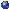## Events

##### GeometryDaniel Douglas, PMA 9.166 : Webs on surfaces
Thursday, September 29, 2022, 03:30pm - 04:30pm
Webs are generalizations of closed curves. More precisely, for a fixed integer n at least 2, an n-web in a topological space X is the continuous image of an oriented n-valent graph such that each vertex is either a source or a sink. Given a matrix group G, a closed curve assigns a number to each group homomorphism r from the fundamental group \pi_1(X) to G, by taking the trace of r along the curve. Similarly, when G=SL(n) is the group of n-by-n matrices with determinant 1, Sikora  defined the "trace" of r along any n-web. In fancier language, an n-web defines a "trace regular function" on the SL(n)-character variety of X (assuming \pi_1(X) is finitely generated). In higher Teichm?ller theory, namely in the setting where X=S is a surface, webs are candidates for so-called higher laminations. In this talk, we will discuss some of this story, in particular connections to deeper ideas in cluster geometry due to Fock, Goncharov, and Shen. This is joint work with Zhe Sun.Location: PMA 9.166